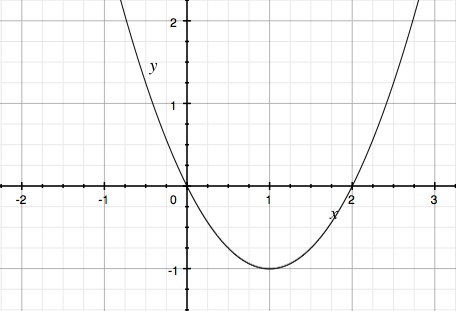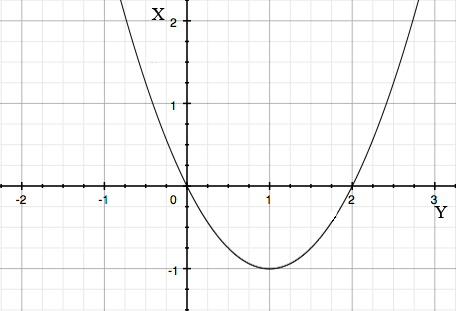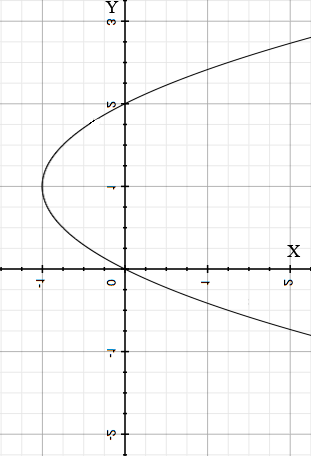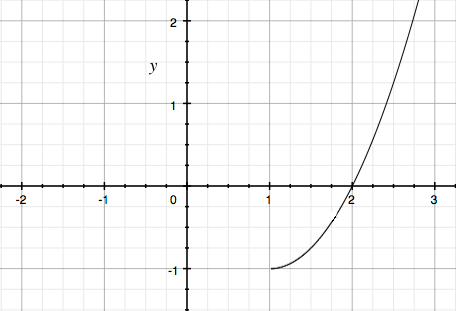SEARCH HOMEMath Central Quandaries & QueriesQuestion from Sasha, a student: Hello, I recently stumbled upon this question and I haven't been able to figure it out. Why is the inverse of y=x(x-2) not a function? Suggest a domain restriction which would ensure that the inverse is a function. Thank You :)Hi Sasha,

I would stat by drawing a graph of $y = x(x-2).$To invert this function graphically you first interchange the roles of $x$ and $y$ by labeling the X-axis as the Y-axis and the Y-axis as the X-axis.Next flip the graph so that the X-axis is horizontal with positive to the right and the Y-axis vertical with positive upwards.This is a graph of the inverse of $y = x(x-2)$ and you can clearly see why it is not a function. For each $x$ value greater than 1 there are two points on the graph with that $x$ value.

The instruction "Suggest a domain restriction which would ensure that the inverse is a function." means to suggest a domain restriction on the original function $y = x(x-2)$ so that the inverse is a function. For example if you define $y = x(x-2)$ for $x \geq 1$ then the graph of this function isand the inverse of this function is a function.

I hope this helps,
PennyMath Central is supported by the University of Regina and the Imperial Oil Foundation.